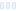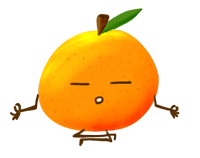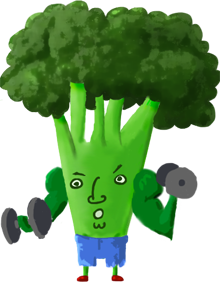# Endurance Formula Thirst Quencher

Main info:

Endurance Formula Thirst Quencher
1 Serving
50 Calories
14 g
0 g
0 g

0 g
0 mg
0 g
200 mg
0 g
0 g

Percent calories from...
Nutrition Facts
For a Serving Size of (g)
How many calories are in Endurance Formula Thirst Quencher? Amount of calories in Endurance Formula Thirst Quencher: Calories Calories from Fat (%)
% Daily Value *
How much fat is in Endurance Formula Thirst Quencher? Amount of fat in Endurance Formula Thirst Quencher: Total Fat
How much sodium is in Endurance Formula Thirst Quencher? Amount of sodium in Endurance Formula Thirst Quencher: Sodium
How much potassium is in Endurance Formula Thirst Quencher? Amount of potassium in Endurance Formula Thirst Quencher: Potassium
How many carbs are in Endurance Formula Thirst Quencher? Amount of carbs in Endurance Formula Thirst Quencher: Carbohydrates
How many net carbs are in Endurance Formula Thirst Quencher? Amount of net carbs in Endurance Formula Thirst Quencher: Net carbs
How much fiber is in Endurance Formula Thirst Quencher? Amount of fiber in Endurance Formula Thirst Quencher: Fiber
How much glucose is in Endurance Formula Thirst Quencher? Amount of glucose in Endurance Formula Thirst Quencher: Glucose
How much protein is in Endurance Formula Thirst Quencher? Amount of protein in Endurance Formula Thirst Quencher: Protein
Vitamins and minerals
How much Vitamin A is in Endurance Formula Thirst Quencher? Amount of Vitamin A in Endurance Formula Thirst Quencher: Vitamin A
How much Vitamin C is in Endurance Formula Thirst Quencher? Amount of Vitamin C in Endurance Formula Thirst Quencher: Vitamin C
How much Calcium is in Endurance Formula Thirst Quencher? Amount of Calcium in Endurance Formula Thirst Quencher: Calcium
How much Iron is in Endurance Formula Thirst Quencher? Amount of Iron in Endurance Formula Thirst Quencher: Iron
Fatty acids
Amino acids
* The Percent Daily Values are based on a 2,000 calorie diet, so your values may change depending on your calorie needs.Loading similar foods...
Note: Any items purchased after clicking our Amazon buttons will give us a little referral bonus. If you do click them, thank you!Be cool

- Zen orangeI never skip arm day

- Buff broccoli